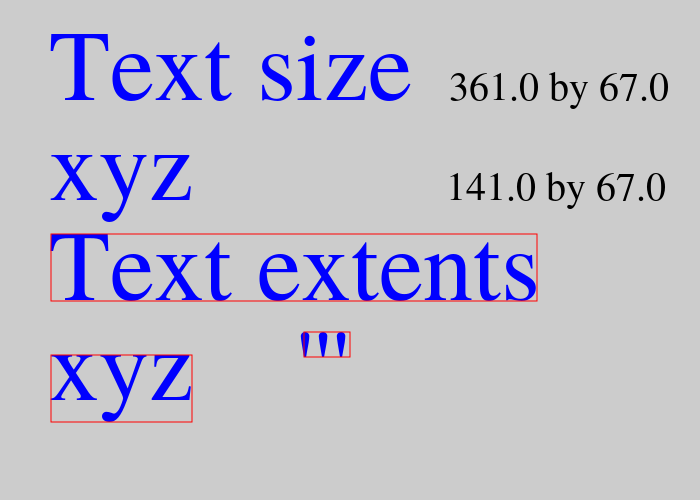# Text metrics in generativepy

Martin McBride, 2022-01-04
Categories generativepy generativepy tutorialThe previous article explains how to use text in generativepy. In this article, we will look at text metrics. Text metrics can be used to find the size and position of the text.

## Text metric methods

Here is some code that draws text and measures its size.

from generativepy.drawing import make_image, setup
from generativepy.color import Color
from generativepy.geometry import Text, Rectangle

def draw(ctx, pixel_width, pixel_height, frame_no, frame_count):
setup(ctx, pixel_width, pixel_width, background=Color(0.8))

x, y = 50, 100
text = Text(ctx).of("Text size", (x, y)).font("Times").size(100).fill(Color('blue'))
width, height = text.get_size()
Text(ctx).of('{} by {}'.format(width, height), (x+400, y))\
.font("Times").size(40).fill(Color('black'))

x, y = 50, 200
text = Text(ctx).of("xyz", (x, y)).font("Times").size(100).fill(Color('blue'))
width, height = text.get_size()
Text(ctx).of('{} by {}'.format(width, height), (x+400, y))\
.font("Times").size(40).fill(Color('black'))

x, y = 50, 300
text = Text(ctx).of("Text extents", (x, y)).font("Times").size(100).fill(Color('blue'))
Rectangle(ctx).of_corner_size((x + x_bearing, y+y_bearing), width, height).stroke(Color('red'))

x, y = 50, 400
text = Text(ctx).of("xyz", (x, y)).font("Times").size(100).fill(Color('blue'))
Rectangle(ctx).of_corner_size((x + x_bearing, y + y_bearing), width, height).stroke(Color('red'))

x, y = 300, 400
text = Text(ctx).of("'''", (x, y)).font("Times").size(100).fill(Color('blue'))
Rectangle(ctx).of_corner_size((x + x_bearing, y + y_bearing), width, height).stroke(Color('red'))

make_image("text-metrics.png", draw, 700, 500)


This code is available on github in tutorial/shapes/text-metrics.py.

Here is the result:## Getting the text size

This code (from the full listing above) draws some text and finds its size:

    x, y = 50, 100
text = Text(ctx).of("Text size", (x, y)).font("Times").size(100).fill(Color('blue'))
width, height = text.get_size()
Text(ctx).of('{} by {}'.format(width, height), (x+400, y))\
.font("Times").size(40).fill(Color('black'))


We draw some text, containing the string "Text size", in the usual way. But we also save the Text object in the variable text.

Next we call text.get_size() to get the size of the text element. This returns a tuple (width, height) that we unpack into two variables.

These give the exact size of the text rectangle. We display this value as text next to the original text. The rectangle is 361 by 67 units. Since we are in default user space, that means that the text box is 361 by 67 pixels.

What exactly is the text box? It is the smallest rectangle that completely encloses the pixels marked by the text string. The width is from the left-hand side of the first 'T' to the right-hand side of the last 'e'. The height is from the baseline of the text up to the top of the 'T' (because that is the tallest character).

We do this again with the string xyz:

    x, y = 50, 200
text = Text(ctx).of("xyz", (x, y)).font("Times").size(100).fill(Color('blue'))
width, height = text.get_size()
Text(ctx).of('{} by {}'.format(width, height), (x+400, y))\
.font("Times").size(40).fill(Color('black'))


This time, the height of the text box is a little different. It goes from the bottom of the tail of the letter 'y' to the top of the 'xyz'.

An important point here is that get_size tells you the size of the text box but it doesn't tell you its position relative to the text itself. For example, the bottom of the text box might be at the baseline, or below the baseline, depending on whether the text contains any characters with descenders.

## Getting the text metrics

We can solve this problem using get_metrics():

    x, y = 50, 300
text = Text(ctx).of("Text extents", (x, y)).font("Times").size(100).fill(Color('blue'))
Rectangle(ctx).of_corner_size((x + x_bearing, y + y_bearing), width, height).stroke(Color('red'))


This function returns 6 values:

• x_bearing, y_bearing - the start position of the text box.
• width, height - the size of the text box (exactly the same as for get_size).
• x_advance, y_advance - the position of the next character.

The x_bearing gives the x-position of the text box relative to the start of the text. The y_bearing gives the y-position of the text box relative to the text baseline.

This means that if you place text at position (x, y), assuming it is horizontal alignment is left and the vertical alignment is baseline, the text box can be drawn with:

    Rectangle(ctx).of_corner_size((x + x_bearing, y + y_bearing), width, height).stroke(Color('red'))


If we wanted to draw a second text string after this one, we can position it by moving along by x_advance in the x-direction. This will position the string correctly, but it will not leave a space between the two strings. y_advance will usually be zero for Western fonts, and can be ignored.

This assumes a left-to-right writing system. In other systems, the advances work differently. For example, in a top-to-bottom writing system, the x_advance will be zero and the y_advance will indicate how far below to place the next string.

The image above shows the text boxes for the strings "Text extents", "xyz", and "'''". Notice that the box is positioned correctly in all cases.

If you found this article useful, you might be interested in the book Computer Graphics in Python or other books by the same author.

#### Popular tags

2d arrays abstract data type alignment and angle animation arange arc array arrays behavioural pattern bezier curve built-in function callable object chain circle classes clipping close closure cmyk colour combinations comparison operator comprehension context context manager conversion count creational pattern data science data types design pattern device space dictionary drawing duck typing efficiency ellipse else encryption enumerate fill filter font font style for loop function function composition function plot functools game development generativepy tutorial generator geometry gif global variable gradient greyscale higher order function hsl html image image processing imagesurface immutable object in operator index inner function input installing iter iterable iterator itertools join l system lambda function len line linear gradient linspace list list comprehension logical operator lru_cache magic method mandelbrot mandelbrot set map matplotlib monad mutability named parameter numeric python numpy object open operator optimisation optional parameter or pandas partial application path pattern permutations polygon positional parameter print pure function python standard library radial gradient range recipes rectangle recursion reduce repeat rgb rotation roundrect scaling scipy sector segment sequence setup shape singleton slice slicing sound spirograph sprite square str stream string stroke structural pattern subpath symmetric encryption template text text metrics tinkerbell fractal transform translation transparency triangle truthy value tuple turtle unpacking user space vectorisation webserver website while loop zip